Hostname: page-component-797576ffbb-lm8cj Total loading time: 0 Render date: 2023-12-07T13:38:40.277Z Has data issue: false Feature Flags: { "corePageComponentGetUserInfoFromSharedSession": true, "coreDisableEcommerce": false, "useRatesEcommerce": true } hasContentIssue false

# Vorticity moments in four numerical simulations of the 3D Navier–Stokes equations

Published online by Cambridge University Press:  04 September 2013

*

## Abstract

The issue of intermittency in numerical solutions of the 3D Navier–Stokes equations on a periodic box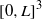${[0, L] }^{3}$ is addressed through four sets of numerical simulations that calculate a new set of variables defined by${D}_{m} (t)= {({ \varpi }_{0}^{- 1} {\Omega }_{m} )}^{{\alpha }_{m} }$ for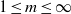$1\leq m\leq \infty$ where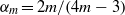${\alpha }_{m} = 2m/ (4m- 3)$ and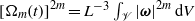${[{\Omega }_{m} (t)] }^{2m} = {L}^{- 3} \int \nolimits _{\mathscr{V}} {\vert \boldsymbol{\omega} \vert }^{2m} \hspace{0.167em} \mathrm{d} V$ with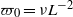${\varpi }_{0} = \nu {L}^{- 2}$. All four simulations unexpectedly show that the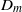${D}_{m}$ are ordered for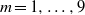$m= 1, \ldots , 9$ such that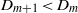${D}_{m+ 1} \lt {D}_{m}$. Moreover, the${D}_{m}$ squeeze together such that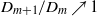${D}_{m+ 1} / {D}_{m} \nearrow 1$ as$m$ increases. The values of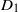${D}_{1}$ lie far above the values of the rest of the${D}_{m}$, giving rise to a suggestion that a depletion of nonlinearity is occurring which could be the cause of Navier–Stokes regularity. The first simulation is of very anisotropic decaying turbulence; the second and third are of decaying isotropic turbulence from random initial conditions and forced isotropic turbulence at fixed Grashof number respectively; the fourth is of very-high-Reynolds-number forced, stationary, isotropic turbulence at up to resolutions of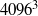$409{6}^{3}$.

## JFM classification

Type
Papers
Information
Journal of Fluid Mechanics , 10 October 2013 , pp. 316 - 331

## Access options

Get access to the full version of this content by using one of the access options below. (Log in options will check for institutional or personal access. Content may require purchase if you do not have access.)

## References

Arneodo, A., Benzi, R., Berg, J., Biferale, L., Bodenschatz, E., Busse, A., Calzavarini, E., Castaing, B., Cencini, M., Chevillard, L., Fisher, R. T., Grauer, R., Homann, H., Lamb, D., Lanotte, A. S., Leveque, E., Luethi, B., Mann, J., Mordant, N., Mueller, W.-C., Ott, S., Ouellette, N. T., Pinton, J.-F., Pope, S. B., Roux, S. G., Toschi, F., Xu, H. & Yeung, P. K. 2008 Universal intermittent properties of particle trajectories in highly turbulent flows. Phys. Rev. Lett. 100, 254504.Google Scholar
Betchov, R. 1956 An inequality concerning the production of vorticity in isotropic turbulence. J. Fluid Mech. 1, 497504.Google Scholar
Boffetta, G., Mazzino, A. & Vulpiani, A. 2008 Twenty-five years of multifractals in fully developed turbulence: a tribute to Giovanni Paladin. J. Phys. A: Math. Theor. 41, 363001.Google Scholar
Bustamante, M. D. & Kerr, R. M. 2008 3D Euler about a 2D symmetry plane. Physica D 237, 19121920.Google Scholar
Chakraborty, S., Frisch, U. & Ray, S. S. 2012 Nelkin scaling for the Burgers equation and the role of high-precision calculations. Phys. Rev. E 85, 015301.Google Scholar
Doering, C. R. & Foias, C. 2002 Energy dissipation in body-forced turbulence. J. Fluid Mech. 467, 289306.Google Scholar
Doering, C. R & Gibbon, J. D. 1995 Applied Analysis of the Navier–Stokes Equations. Cambridge University Press.Google Scholar
Donzis, D., Sreenivasan, K. & Yeung, P. K. 2012 Some results on the Reynolds number scaling of pressure statistics in isotropic turbulence. Physica D 241, 164168.Google Scholar
Donzis, D. & Yeung, P. K. 2010 Resolution effects and scaling in numerical simulations of passive scalar mixing in turbulence. Physica D 239, 12781287.Google Scholar
Donzis, D., Yeung, P. K. & Sreenivasan, K. 2008 Dissipation and enstrophy in isotropic turbulence: scaling and resolution effects in direct numerical simulations. Phys. Fluids 20, 045108.Google Scholar
Eswaran, V. & Pope, S. B. 1988 An examination of forcing in direct numerical simulations of turbulence. Comput. Fluids 16, 257278.Google Scholar
Frisch, U. 1995 Turbulence: the Legacy of A. N. Kolmogorov. Cambridge University Press.Google Scholar
Gibbon, J. D. 2010 Regularity and singularity in solutions of the three-dimensional Navier–Stokes equations. Proc. R. Soc. A 466, 25872604.Google Scholar
Gibbon, J. D. 2011 A hierarchy of length scales for weak solutions of the three-dimensional Navier–Stokes equations. Comm. Math. Sci. 10, 131136.Google Scholar
Gibbon, J. D. 2012 Conditional regularity of solutions of the three-dimensional Navier–Stokes equations and implications for intermittency. J. Math. Phys. 53, 115608.Google Scholar
Gibbon, J. D. 2013 Dynamics of scaled vorticity norms for the three-dimensional Navier–Stokes and Euler equations. Procedia IUTAM: Proceedings of IUTAM Symposium Topological Fluid Dynamics II, vol. 7, pp 39–48.Google Scholar
Hentschel, H. G. E. & Procaccia, I. 1983 The infinite number of generalized dimensions of fractals and strange attractors. Physica D 8, 435444.Google Scholar
Holm, D. D. & Kerr, R. M. 2007 Helicity in the formation of turbulence. Phys. Fluids 19, 025101.Google Scholar
Ishihara, T., Gotoh, T. & Kaneda, Y. 2009 Study of high-Reynolds number isotropic turbulence by direct numerical simulation. Annu. Rev. Fluid Mech. 41, 16180.Google Scholar
Jimenez, J., Wray, A., Saffman, P. G. & Rogallo, R. S. 1993 The structure of intense vorticity in isotropic turbulence. J. Fluid Mech. 255, 6590.Google Scholar
Kerr, R. M. 1985 Higher-order derivative correlations and the alignment of small-scale structures in isotropic numerical turbulence. J. Fluid Mech. 153, 3158.Google Scholar
Kerr, R. M. 1993 Evidence for a singularity of the three-dimensional incompressible Euler equations. Phys. Fluids A 5, 17251746.Google Scholar
Kerr, R. M. 2011 Vortex stretching as a mechanism for quantum kinetic energy decay. Phys. Rev. Lett. 106, 224501.Google Scholar
Kerr, R. M. 2012 Dissipation and enstrophy statistics in turbulence: are the simulations and mathematics converging? J. Fluid Mech. 700, 14.Google Scholar
Kerr, R. M. 2013 Bounds on a singular attractor in Euler using vorticity moments. Procedia IUTAM, Proceedings of IUTAM Symposium Topological Fluid Dynamics II, vol 7, pp. 49–58.Google Scholar
Kerr, R. M. 2013a Swirling, turbulent vortex rings formed from a chain reaction of reconnection events. Phys. Fluids 25, 065101.Google Scholar
Kerr, R. M. 2013b Bounds for Euler from vorticity moments and line divergence. J. Fluid Mech. 729, R2.Google Scholar
Meneveau, C. & Sreenivasan, K. R. 1991 The multifractal nature of turbulent energy dissipation. J. Fluid Mech. 224, 429484.Google Scholar
Meneveau, C., Sreenivasan, K. R., Kailasnath, P. & Fan, M. S. 1990 Joint multifractal measures: theory and applications to turbulence. Phys. Rev. A 41, 894913.Google Scholar
Nelkin, M. 1990 Multifractal scaling of velocity derivatives in turbulence. Phys. Rev. A 42, 72267229.Google Scholar
Orszag, S. A. & Patterson, G. S. 1972 Numerical simulation of three-dimensional homogeneous isotropic turbulence. Phys. Rev. Lett. 28, 7679.Google Scholar
Pandit, R., Perlekar, P. & Ray, S. S. 2009 Statistical properties of turbulence: an overview. Pramana – Journal of Physics 73, 157191.Google Scholar
Ray, S. S., Mitra, D. & Pandit, R. 2008 The universality of dynamic multiscaling in homogeneous, isotropic Navier–Stokes and passive-scalar turbulence. New J. Phys. 10, 033003.Google Scholar
Ray, S. S., Mitra, D., Perlekar, P. & Pandit, R. 2011 Dynamic multiscaling in two-dimensional fluid turbulence. Phys. Rev. Lett. 107, 184503.Google Scholar
Rogallo, R. S. 1981 Numerical experiments in homogeneous turbulence. Tech. Mem. 81835. NASA.Google Scholar
Sahoo, G., Perlekar, P. & Pandit, R. 2011 Systematics of the magnetic-Prandtl-number dependence of homogeneous, isotropic magnetohydrodynamic turbulence. New J. Phys. 13, 013036.Google Scholar
Schumacher, J., Sreenivasan, K. R. & Yakhot, V. 2007 Asymptotic exponents from low-Reynolds-number flows. New J. Phys. 9, 89107.Google Scholar
Sreenivasan, K. R. 1985 On the fine-scale intermittency of turbulence. J. Fluid Mech. 151, 81103.Google Scholar
Yakhot, V. & Sreenivasan, K. R. 2004 Towards a dynamical theory of multifractals in turbulence. Physica A 343, 147155.Google Scholar
Yeung, P. K., Donzis, D. & Sreenivasan, K. R. 2012 Dissipation, enstrophy and pressure statistics in turbulence simulations at high Reynolds numbers. J. Fluid Mech. 700, 515.Google Scholar